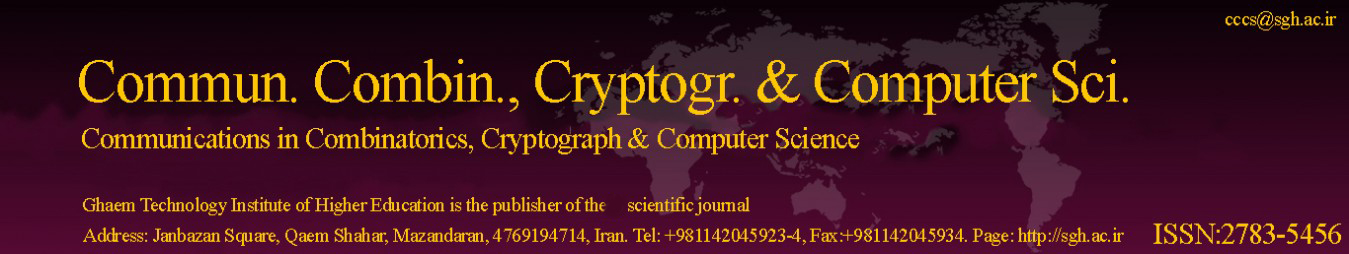Original Research Article

Article volume = 2022 and issue = 1

Pages: 90–93

Article publication Date: November 21, 2022

# Some Conjectures on Average of Fibonacci and Lucas Sequences

#### Daniel Yaqubi(a) and Amirali Fatehizadeh(b)

(a) Department of Computer Science, University of Torbat e Jam, Iran.

(b) Department of Mathematical Sciences, Sharif University of Technology, Tehran, Iran.

##### Abstract:

The arithmetic mean of the first n Fibonacci numbers is not an integer for all n. However, for some values of n we can observe that it is an integer. In this paper we consider the sequence of integers n for that the average of the first n Fibonacci numbers is an integer. We prove some interesting properties and present two related conjectures.

##### Keywords:

Fibonacci number, Lucas number, Pisano period, rank of appearance, restricted period.

##### References:
•  T. M. Apostol, Introduction to Analytic Number Theory, Springer, 1998. 2
•  J. Fulton and W. Morris, On arithmetical functions related to the Fibonacci numbers, Acta Arith. 16 (1969), 105–110. 2, 2, 2.2
•  D. E. Knuth, The Art of Computer Programming, Addison–Wesley, 1968. 1
•  T. Koshy, Fibonacci and Lucas Numbers with Applications, New York, NY: John Wiley and Sons, 2001. 1, 2, 2
•  J. L. Lagrange Serret, Oeuvres de Lagrange, Gauthier-Villars, 1882. 1, 2
•  T. Lengyel, The order of Fibonacci and Lucas numbers, Fibonacci Quart. 33 (1995), 234–239. 2, 2.6
•  P. Ribenboim, The Little Book of Big Primes, Springer, 1991. 1
•  D. W. Robinson, The Fibonacci Matrix Modulo m, Fibonacci Quart. 1 (1963), 29–36. 1, 2, 2
•  OEIS Foundation Inc., The On-Line Encyclopedia of Integer Sequences, 2021. 1, 2
•  L. Somer and M. Krizek, Fixed points and upper bounds for the rank of appearance in Lucas sequences, Fibonacci Quart. 51 (2013), 291–306. 2
•  D. D. Wall Fibonacci series modulo m, Amer. Math. Monthly 67 (1960), 525–532. 1